# smoothdata

Smooth noisy data

## Syntax

``B = smoothdata(A)``
``B = smoothdata(A,dim)``
``B = smoothdata(___,method)``
``B = smoothdata(___,method,window)``
``B = smoothdata(___,nanflag)``
``B = smoothdata(___,Name,Value)``
``````[B,window] = smoothdata(___)``````

## Description

example

````B = smoothdata(A)` returns a moving average of the elements of a vector using a fixed window length that is determined heuristically. The window slides down the length of the vector, computing an average over the elements within each window. If `A` is a matrix, then `smoothdata` computes the moving average down each column of `A`.If `A` is a multidimensional array, then `smoothdata` operates along the first dimension of `A` whose size does not equal 1.If `A` is a table or timetable with numeric variables, then `smoothdata` operates on each variable of `A` separately. ```

example

````B = smoothdata(A,dim)` specifies the dimension of `A` to operate along. For example, if `A` is a matrix, then `smoothdata(A,2)` smooths the data in each row of `A`.```

example

````B = smoothdata(___,method)` specifies the smoothing method for either of the previous syntaxes. For example, `smoothdata(A,'sgolay')` uses a Savitzky-Golay filter to smooth the data in `A`.```

example

````B = smoothdata(___,method,window)` specifies the length of the window used by the smoothing method. For example, `smoothdata(A,'movmedian',5)` smooths the data in `A` by taking the median over a five-element sliding window.```

example

````B = smoothdata(___,nanflag)` specifies how `NaN` values are treated for any of the previous syntaxes. `'omitnan'` ignores `NaN` values and `'includenan'` includes them when computing within each window.```

example

````B = smoothdata(___,Name,Value)` specifies additional parameters for smoothing using one or more name-value arguments. For example, if `t` is a vector of time values, then `smoothdata(A,'SamplePoints',t)` smooths the data in `A` relative to the times in `t`.```

example

``````[B,window] = smoothdata(___)``` also returns the moving window length.```

## Examples

collapse all

Create a vector containing noisy data, and smooth the data with a moving average. Plot the original and smoothed data.

```x = 1:100; A = cos(2*pi*0.05*x+2*pi*rand) + 0.5*randn(1,100); B = smoothdata(A); plot(x,A,'-o',x,B,'-x') legend('Original Data','Smoothed Data')```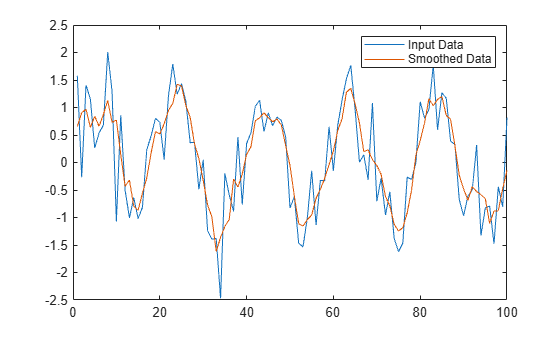Create a matrix whose rows represent three noisy signals. Smooth the three signals using a moving average, and plot the smoothed data.

```x = 1:100; s1 = cos(2*pi*0.03*x+2*pi*rand) + 0.5*randn(1,100); s2 = cos(2*pi*0.04*x+2*pi*rand) + 0.4*randn(1,100) + 5; s3 = cos(2*pi*0.05*x+2*pi*rand) + 0.3*randn(1,100) - 5; A = [s1; s2; s3]; B = smoothdata(A,2); plot(x,B(1,:),x,B(2,:),x,B(3,:))```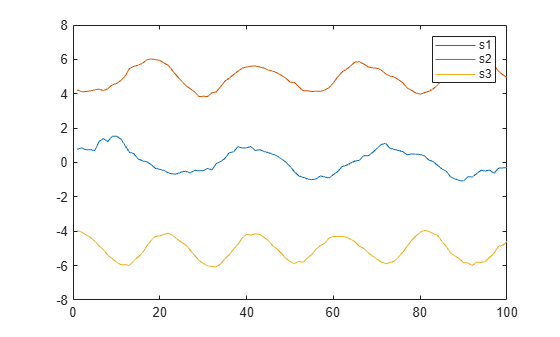Smooth a vector of noisy data with a Gaussian-weighted moving average filter. Display the window length used by the filter.

```x = 1:100; A = cos(2*pi*0.05*x+2*pi*rand) + 0.5*randn(1,100); [B,window] = smoothdata(A,'gaussian'); window```
```window = 4 ```

Smooth the original data with a larger window of length 20. Plot the smoothed data for both window lengths.

```C = smoothdata(A,'gaussian',20); plot(x,B,'-o',x,C,'-x') legend('Small Window','Large Window')```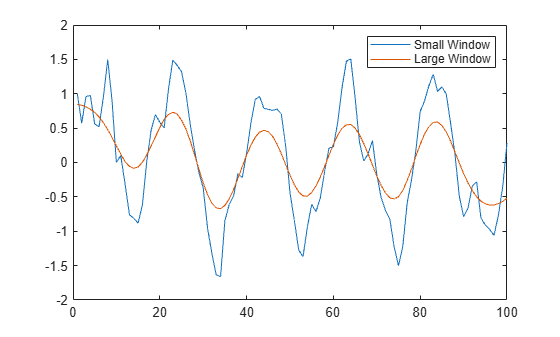Create a noisy vector containing `NaN` values, and smooth the data ignoring `NaN`, which is the default.

```A = [NaN randn(1,48) NaN randn(1,49) NaN]; B = smoothdata(A);```

Smooth the data including `NaN` values. The average in a window containing `NaN` is `NaN`.

`C = smoothdata(A,'includenan');`

Plot the smoothed data in `B` and `C`.

```plot(1:100,B,'-o',1:100,C,'-x') legend('Ignore NaN','Include NaN')```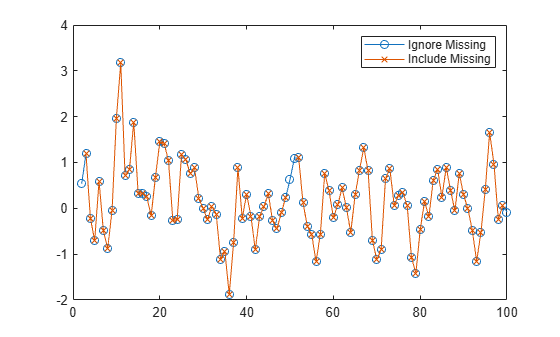Create a vector of noisy data that corresponds to a time vector `t`. Smooth the data relative to the times in `t`, and plot the original data and the smoothed data.

```x = 1:100; A = cos(2*pi*0.05*x+2*pi*rand) + 0.5*randn(1,100); t = datetime(2017,1,1,0,0,0) + hours(0:99); B = smoothdata(A,'SamplePoints',t); plot(t,A,'-o',t,B,'-x') legend('Original Data','Smoothed Data')```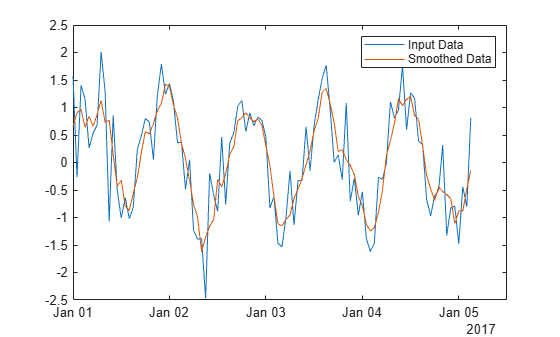## Input Arguments

collapse all

Input data, specified as a vector, matrix, multidimensional array, table, or timetable. If `A` is a table or timetable, then either the variables must be numeric, or you must use the `DataVariables` name-value argument to list numeric variables explicitly. Specifying variables is useful when you are working with a table that also contains nonnumeric variables.

Data Types: `double` | `single` | `int8` | `int16` | `int32` | `int64` | `uint8` | `uint16` | `uint32` | `uint64` | `logical` | `table` | `timetable`

Complex Number Support: Yes

Operating dimension, specified as a positive integer scalar. If no value is specified, then the default is the first array dimension whose size does not equal 1.

Consider an `m`-by-`n` input matrix, `A`:

• `smoothdata(A,1)` smooths the data in each column of `A` and returns an `m`-by-`n` matrix.• `smoothdata(A,2)` smooths the data in row of `A` and returns an `m`-by-`n` matrix.For table or timetable input data, `dim` is not supported and operation is along each table or timetable variable separately.

Smoothing method, specified as one of these values:

• `'movmean'` — Moving average over each window of `A`. This method is useful for reducing periodic trends in data.

• `'movmedian'` — Moving median over each window of `A`. This method is useful for reducing periodic trends in data when outliers are present.

• `'gaussian'` — Gaussian-weighted moving average over each window of `A`.

• `'lowess'` — Linear regression over each window of `A`. This method can be computationally expensive, but results in fewer discontinuities.

• `'loess'` — Quadratic regression over each window of `A`. This method is slightly more computationally expensive than `'lowess'`.

• `'rlowess'` — Robust linear regression over each window of `A`. This method is a more computationally expensive version of the method `'lowess'`, but it is more robust to outliers.

• `'rloess'` — Robust quadratic regression over each window of `A`. This method is a more computationally expensive version of the method `'loess'`, but it is more robust to outliers.

• `'sgolay'` — Savitzky-Golay filter, which smooths according to a quadratic polynomial that is fitted over each window of `A`. This method can be more effective than other methods when the data varies rapidly.

Window length, specified as a positive integer scalar, a two-element vector of positive integers, a positive duration scalar, or a two-element vector of positive durations.

When `window` is a positive integer scalar, then the window is centered about the current element and contains `window-1` neighboring elements. If `window` is even, then the window is centered about the current and previous elements.

When `window` is a two-element vector of positive integers `[b f]`, the window contains the current element, `b` elements backward, and `f` elements forward.

When `A` is a timetable or `SamplePoints` is specified as a `datetime` or `duration` vector, `window` must be of type `duration`, and the window is computed relative to the sample points.

When the window length is also specified as an output argument, the output value matches the input value.

`NaN` condition, specified as one of these values:

• `'omitnan'` — Ignore `NaN` values in the input. If a window contains all `NaN` values, then `smoothdata` returns `NaN`.

• `'includenan'` — Include `NaN` values when computing within each window, resulting in `NaN`.

### Name-Value Arguments

Specify optional pairs of arguments as `Name1=Value1,...,NameN=ValueN`, where `Name` is the argument name and `Value` is the corresponding value. Name-value arguments must appear after other arguments, but the order of the pairs does not matter.

Before R2021a, use commas to separate each name and value, and enclose `Name` in quotes.

Example: `smoothdata(A,'SmoothingFactor',0.5)`

Data Options

collapse all

Sample points, specified as a vector of sample point values or one of the options in the following table when the input data is a table. The sample points represent the x-axis locations of the data, and must be sorted and contain unique elements. Sample points do not need to be uniformly sampled. The vector ```[1 2 3 ...]``` is the default.

When the input data is a table, you can specify the sample points as a table variable using one of these options:

Option for Table InputDescriptionExamples
Variable name

A character vector or scalar string specifying a single table variable name

`'Var1'`

`"Var1"`

Scalar variable index

A scalar table variable index

`3`

Logical vector

A logical vector whose elements each correspond to a table variable, where `true` specifies the corresponding variable as the sample points, and all other elements are `false`

`[true false false]`

Function handle

A function handle that takes a table variable as input and returns a logical scalar, which must be `true` for only one table variable

`@isnumeric`

`vartype` subscript

A table subscript generated by the `vartype` function that returns a subscript for only one variable

`vartype('numeric')`

Note

This name-value argument is not supported when the input data is a `timetable`. Timetables use the vector of row times as the sample points. To use different sample points, you must edit the timetable so that the row times contain the desired sample points.

Moving windows are defined relative to the sample points. For example, if `t` is a vector of times corresponding to the input data, then `smoothdata(rand(1,10),3,'SamplePoints',t)` has a window that represents the time interval between `t(i)-1.5` and `t(i)+1.5`.

When the sample points vector has data type `datetime` or `duration`, the moving window length must have type `duration`.

Example: `smoothdata(A,'SamplePoints',0:0.1:10)`

Example: `smoothdata(T,'SamplePoints',"Var1")`

Data Types: `double` | `single` | `datetime` | `duration`

Table variables to operate on, specified as one of the options in this table. The `DataVariables` value indicates which variables of the input table to smooth.

Other variables in the table not specified by `DataVariables` pass through to the output without being smoothed.

OptionDescriptionExamples
Variable name

A character vector or string scalar specifying a single table variable name

`'Var1'`

`"Var1"`

Vector of variable names

A cell array of character vectors or string array, where each element is a table variable name

`{'Var1' 'Var2'}`

`["Var1" "Var2"]`

Scalar or vector of variable indices

A scalar or vector of table variable indices

`1`

`[1 3 5]`

Logical vector

A logical vector whose elements each correspond to a table variable, where `true` includes the corresponding variable and `false` excludes it

`[true false true]`

Function handle

A function handle that takes a table variable as input and returns a logical scalar

`@isnumeric`

`vartype` subscript

A table subscript generated by the `vartype` function

`vartype("numeric")`

Example: ```smoothdata(T,'DataVariables',["Var1" "Var2" "Var4"])```

Replace values indicator, specified as one of these values when `A` is a table or timetable:

• `true` or `1` — Replace input table variables with table variables containing smoothed data.

• `false` or `0` — Append input table variables with table variables containing smoothed data.

For vector, matrix, or multidimensional array input data, `ReplaceValues` is not supported.

Example: `smoothdata(T,'ReplaceValues',false)`

Smoothing Options

collapse all

Window size factor, specified as a scalar ranging from 0 to 1. Generally, the value of `SmoothingFactor` adjusts the level of smoothing by scaling the heuristic window size. Values near 0 produce smaller moving window lengths, resulting in less smoothing. Values near 1 produce larger moving window lengths, resulting in more smoothing. In some cases, depending on the input data from which the heuristic window size is determined, the value of `SmoothingFactor` may not have a significant impact on the window size used by `smoothdata`.

`SmoothingFactor` is 0.25 by default and can only be specified when `window` is not specified.

Savitzky-Golay degree, specified as a nonnegative integer. This name-value argument can only be specified when `'sgolay'` is the specified smoothing method. The value of `Degree` corresponds to the degree of the polynomial in the Savitzky-Golay filter that fits the data within each window, which is 2 by default.

The value of `Degree` must be less than the window length for uniform sample points. For nonuniform sample points, the value must be less than the maximum number of points in any window.

## Output Arguments

collapse all

Smoothed data, returned as a vector, matrix, multidimensional array, table, or timetable.

`B` is the same size as `A` unless the value of `ReplaceValues` is `false`. If the value of `ReplaceValues` is `false`, then the width of `B` is the sum of the input data width and the number of data variables specified.

Window length, returned as a positive integer scalar, a two-element vector of positive integers, a positive duration scalar, or a two-element vector of positive durations.

When `window` is specified as an input argument, the output value matches the input value. When `window` is not specified as an input argument, then its value is the scalar heuristically determined by `smoothdata` based on the input data.

## Algorithms

When the window size for the smoothing method is not specified, `smoothdata` computes a default window size based on a heuristic. For a smoothing factor τ, the heuristic estimates a moving average window size that attenuates approximately 100*τ percent of the energy of the input data.

## Version History

Introduced in R2017a

expand all